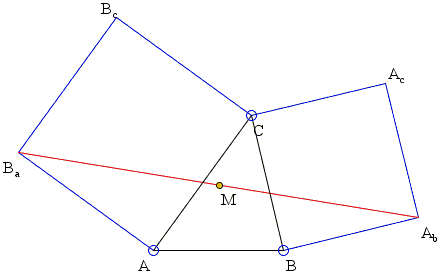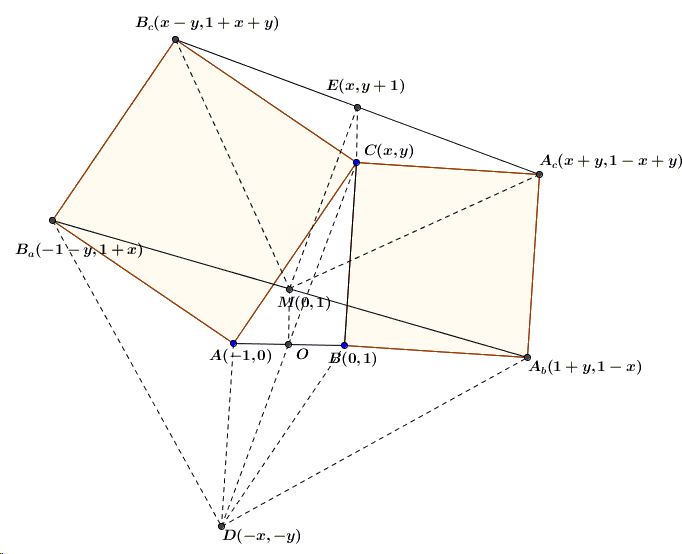# Analytic Proof of Bottema's Theorem

Consider a configuration of two squares ACBcBa and BCAcAb with a common vertex C.Bottema's theorem claims that the midpoint M of the segment AbBa is independent of C.

### Proof

A short analytic proof by Hubert Shutrick demonstrates how simple the theorem actually is.

If $A = (-1,0)$, $B = (1,0)$ and $C = (x,y)$, then $A_b = (y+1, 1-x)$, $B_a = (-1-y, 1+x)$ and their midpoint is $(A_{b} + B_{a})/2 = (0,1)$.

Moreover, the proof makes it rather obvious that the angle $AMB$ is right and, therefore, is the center of the square erected on $AB$ on the side of $C$.

As a practice in analytic geometry we may elaborate on the above diagram:As Hubert Shutrick has observed, $\triangle A_{c}MB_{c}$ is right isosceles. He also noticed that $OECM$, where $E$ is the midpoint of $A_{c}B_{c}$, is a parallelogram. Analytic geometry helps confirm another fact, the one noticed by William McWorter, viz., $\triangle A_{b}B_{a}D$ is also right isosceles.### Bottema's Theorem